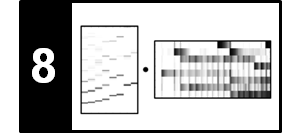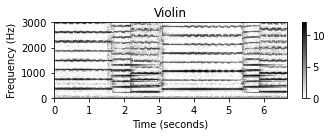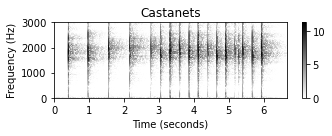# Harmonic–Percussive Separation (HPS)

Following Section 8.1.1 of [Müller, FMP, Springer 2015], we cover in this notebook a basic approach for separating a signal into a harmonic and a percussive component. This approach was originally introduced by Derry FitzGerald:

• Derry FitzGerald: Harmonic/Percussive Separation Using Median Filtering. Proceedings of the International Conference on Digital Audio Effects (DAFx), Graz, Austria, 2010, pp. 246–253.

## Harmonic and Percussive Sounds¶

Musical sounds can comprise a wide range of sound components with different acoustic qualities. In particular, we consider two broad categories of sounds: harmonic sounds and percussive sounds. Loosely speaking, a harmonic sound is what we perceive as pitched sound, what makes us hear melodies and chords. The prototype of a harmonic sound is the acoustic realization of a sinusoid, which corresponds to a horizontal line in a spectrogram representation. The sound of a violin is another typical example of what we consider a harmonic sound. Again, most of the observed structures in the spectrogram are of horizontal nature (even though they are intermingled with noise-like components). On the other hand, a percussive sound is what we perceive as a clash, a knock, a clap, or a click. The sound of a drum stroke or a transient that occurs in the attack phase of a musical tone are further typical examples. The prototype of a percussive sound is the acoustic realization of an impulse, which corresponds to a vertical line in a spectrogram representation.

In the following example, we show the spectrogram representations (using logarithmic compression) of a violin recording, a recording of castanets, and a superposition of these two recordings. For the violin sound one can observe horizontal lines that are stacked on top of each other, which correspond to the harmonics, the integer multiples of the fundamental frequency of a played note.

In :
import os, sys
import numpy as np
from scipy import signal
import matplotlib.pyplot as plt
import IPython.display as ipd
import librosa.display
import soundfile as sf
sys.path.append('..')
import libfmp.b

%matplotlib inline

def compute_plot_spectrogram(x, Fs=22050, N=4096, H=2048, ylim=None,
figsize =(5, 2), title='', log=False):
N, H = 1024, 512
X = librosa.stft(x, n_fft=N, hop_length=H, win_length=N, window='hann',
Y = np.abs(X)**2
if log:
Y_plot = np.log(1 + 100 * Y)
else:
Y_plot = Y
libfmp.b.plot_matrix(Y_plot, Fs=Fs/H, Fs_F=N/Fs, title=title, figsize=figsize)
if ylim is not None:
plt.ylim(ylim)
plt.tight_layout()
plt.show()
return Y

Fs = 22050
fn_wav = os.path.join('..', 'data', 'C8', 'FMP_C8_F02_Long_Violin.wav')
Y = compute_plot_spectrogram(x, Fs=Fs, title = 'Violin', ylim=[0, 3000], log=1)
ipd.display(ipd.Audio(data=x, rate=Fs))

fn_wav = os.path.join('..', 'data', 'C8', 'FMP_C8_F02_Long_Castanets.wav')